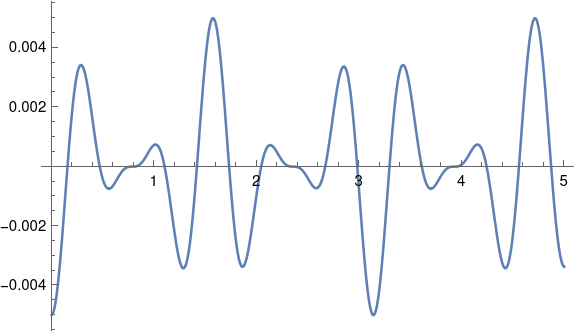# FM signal approximation

FM radio transmits a signal by perturbing (modulating) the frequency of a carrier wave. If the carrier has frequency ω and the signal has frequency q, then the FM signal is

cos(ωt + β cos(qt)).

To understand the modulated signal, it’s useful to write it as a sum of simple sines and cosines with no modulation. I wrote about how to do this exactly using Bessel functions. Today I’ll write about an approximation that’s easier to understand and work with, assuming the modulation index β is small.

Here’s the approximation:

cos(ωt + β cos(qt)) ≈ cos ωt + ½ β ( sin (ω + q)t + sin (ω – q)t ).

This says that to a good approximation, the modulation term adds two sine waves to the carrier, one that adds the signal frequency to the carrier frequency and one that subtracts it.

To establish the approximation and see how the error depends on β, subtract the right side from the left and expand as a Taylor series in β. The first non-zero term in the series is

-½ cos(qt)² cos(ωt) β²

and so if β is small, the approximation error is very small. For example, if β = 0.1, then the approximation error is on the order of 0.005.

As an example, let ω = 10, q = 2, and β = 0.1. Then

cos(10t + 0.1 cos 2t) ≈ cos 10t + 0.05 ( sin 12t + sin 8t )

and the approximation error is plotted below.As predicted, the amplitude of the error is around 0.005, while the amplitude of the FM signal is 1.

## One thought on “FM signal approximation”

1. “If the carrier has frequency ω and the signal has frequency q, then the FM signal is cos(ωt + β cos(qt)).”

Need real-world example, please. Suppose ω = 100 MHz (conveniently in the middle of the FM broadcast band) and q = 1000 Hz (very roughly C two ledger lines above the treble-clef staff). Then according to the regulatory regime of North American broadcasting (and probably lots of other places too), instantaneous ω varies continuously within a range that must not exceed 99925 KHz to 100075 KHz, in other words ω – Δω is proportional to the instantaneous amplitude of the signal, scaled to a maximum absolute value of 75KHz. The reason why FM broadcast engineers need to learn Bessel functions is in order to do a quick slide-rule calculation to reassure themselves that they are not violating FCC regulations. How do we get from your formulation to Δω , which is what matters?